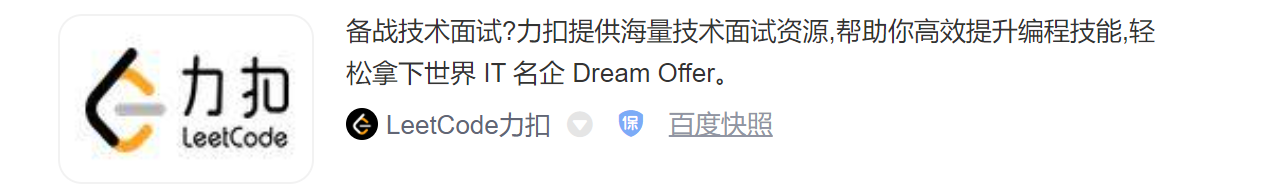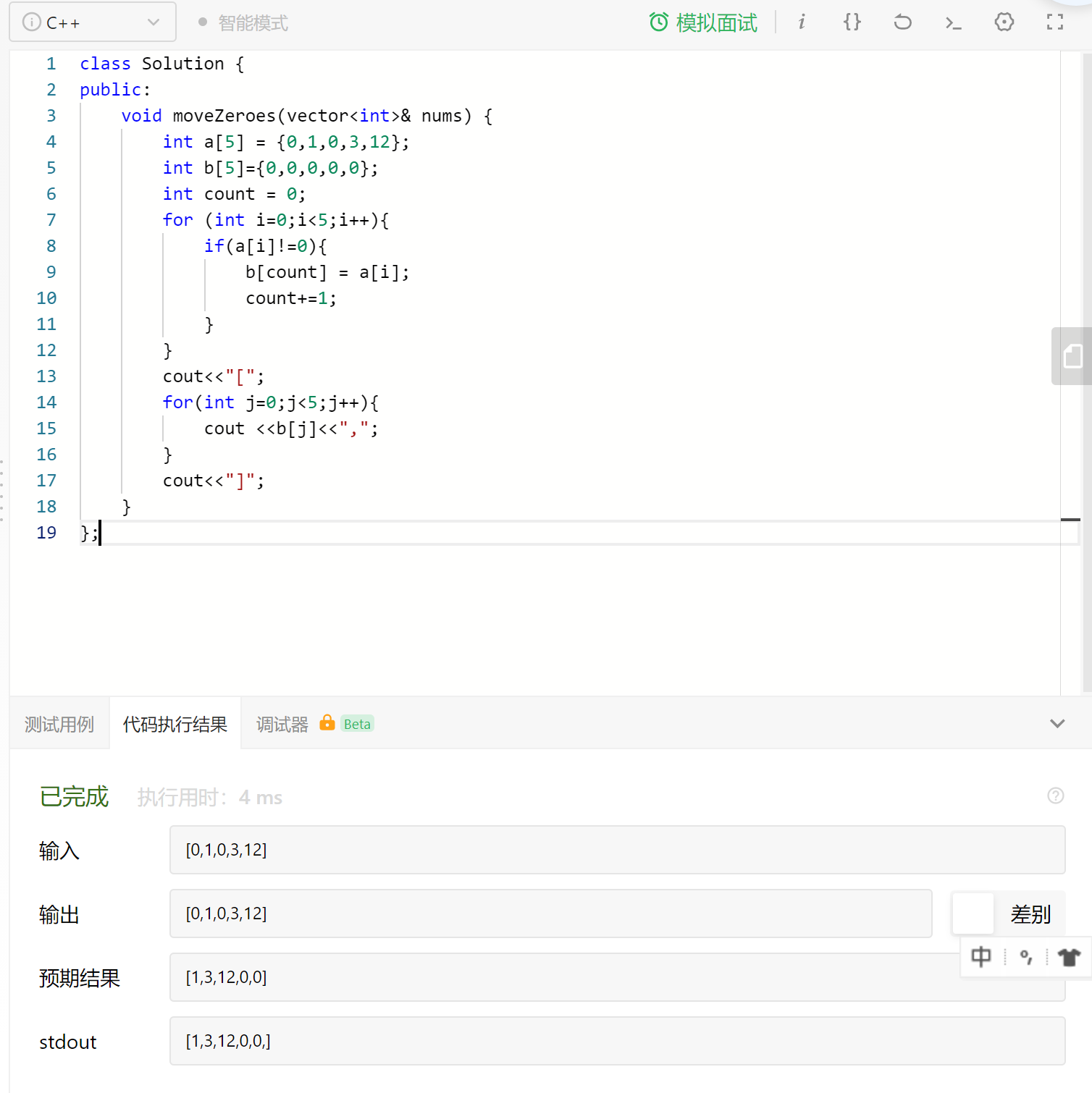力扣（Leetcode）练习 -- 给定一个数组 nums，编写一个函数将所有 0 移动到数组的末尾，同时保持非零元素的相对顺序Wynne方法一：

#include <iostream>using namespace std;int main() {    //定义原数组    int nums={0,1,0,3,12};	int i = 0,j = 0;	//在数组中循环，当数字为非0时，将此数字赋值给数组第一位nums	//循环到第二个非0数字时，赋值给数组第二位nums	//j控制数组的赋值位，赋值后j自动加一，以便下一次的循环赋值    for(i = 0 ; i < 5; i++)    {        if(nums[i] != 0)        {            nums[j++] = nums[i];        }    }    //当循环完非0数字后，将所剩的位置，全部置0    while(j < 5)    {        nums[j++] = 0;    }    //暂时未找到输出数组的好方法，只能用循环来取数字并加格式[,,]    cout<<"[";    for(int k=0;k<5;k++){        cout<<nums[k]<<",";            }    cout<<"]";}

方法二:

#include <iostream>using namespace std;int main() {    //定义两个数组，一个原数组，一个空数组，最终输出的是数组b（原题目中的nums）    int a = {0,1,0,3,12};    int b={0,0,0,0,0};    //count位用来标记数组b中的位置    int count = 0;    //数组a中循环，如果是非0，就提取出来，依次放在数组b中    for (int i=0;i<5;i++){        if(a[i]!=0){	        b[count] = a[i];	        count+=1;	    }    }    //原本只能输出一堆数字，为了保持数组的格式，手动加了[]和，    cout<<"[";	for(int j=0;j<5;j++){	   cout <<b[j]<<",";	}    cout<<"]";}

1）C++在线编译器结果：2）力扣（Leetcode）运行结果：ps.尚未找到输出数组（带格式）的好方法，如果有合适方法，希望能告知~评论 (1 条评论)//定义原数组
int nums={0,1,0,3,12};
int i = 0,j = 0;
//在数组中循环，当数字为非0时，将此数字赋值给数组第一位nums
//循环到第二个非0数字时，赋值给数组第二位nums
//j控制数组的赋值位，赋值后j自动加一，以便下一次的循环赋值
for(i = 0 ; i < 5; i++)
{
if(nums[i] != 0)
{
nums[j++] = nums[i];
}
}
//当循环完非0数字后，将所剩的位置，全部置0
while(j < 5)
{
nums[j++] = 0;
}
//暂时未找到输出数组的好方法，只能用循环来取数字并加格式[,,]
cout<<"[";
for(int k=0,m=0;k<5;k++){
cout< //int m=0;
if(m<4){
cout<<",";
m+=1;
}
}
cout<<"]";

2020 年 11 月 20 日 09:15